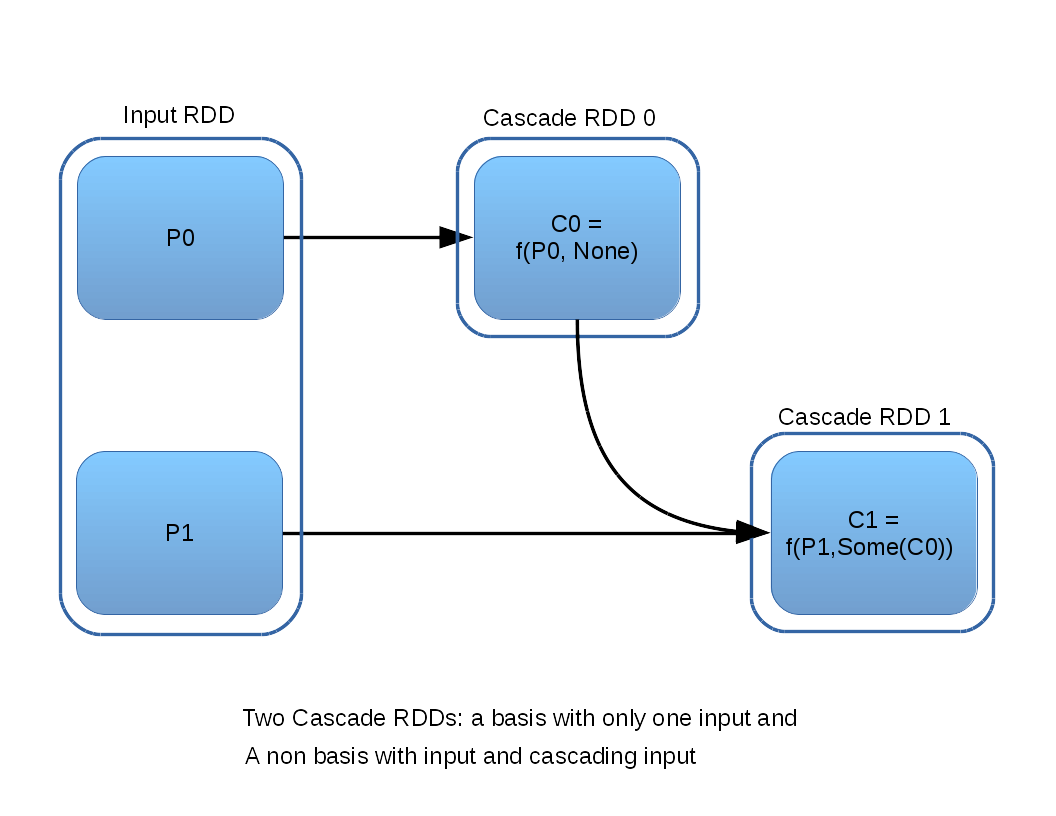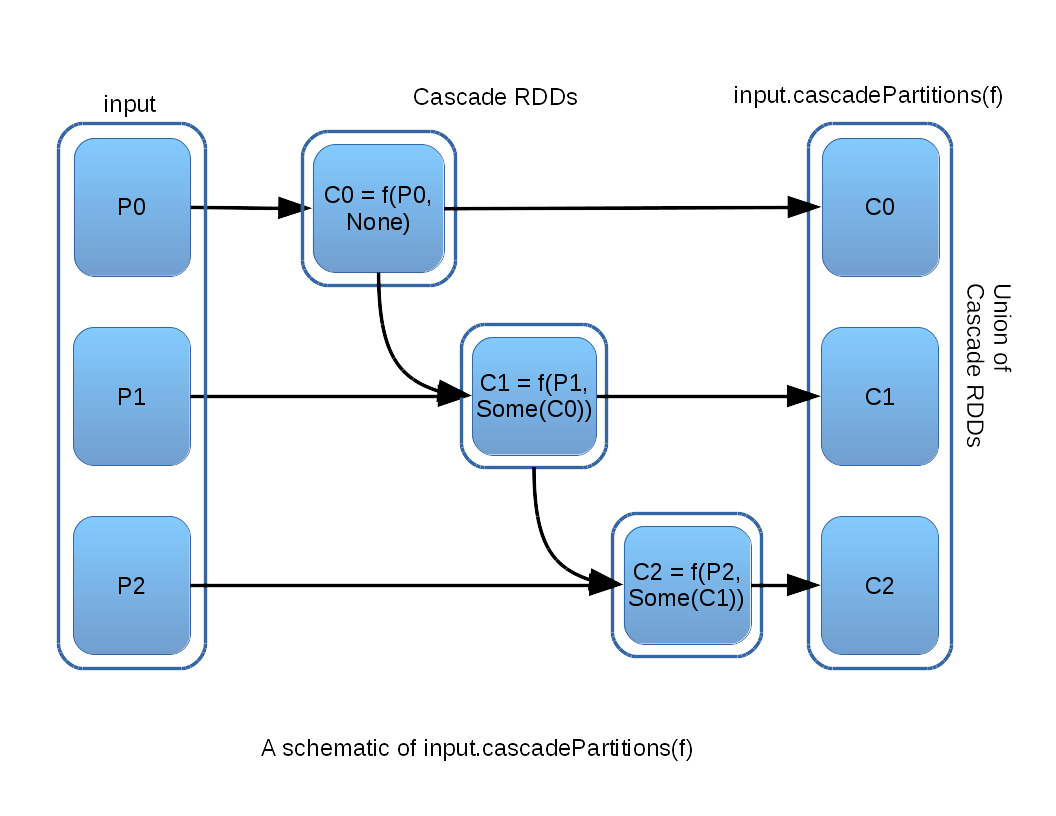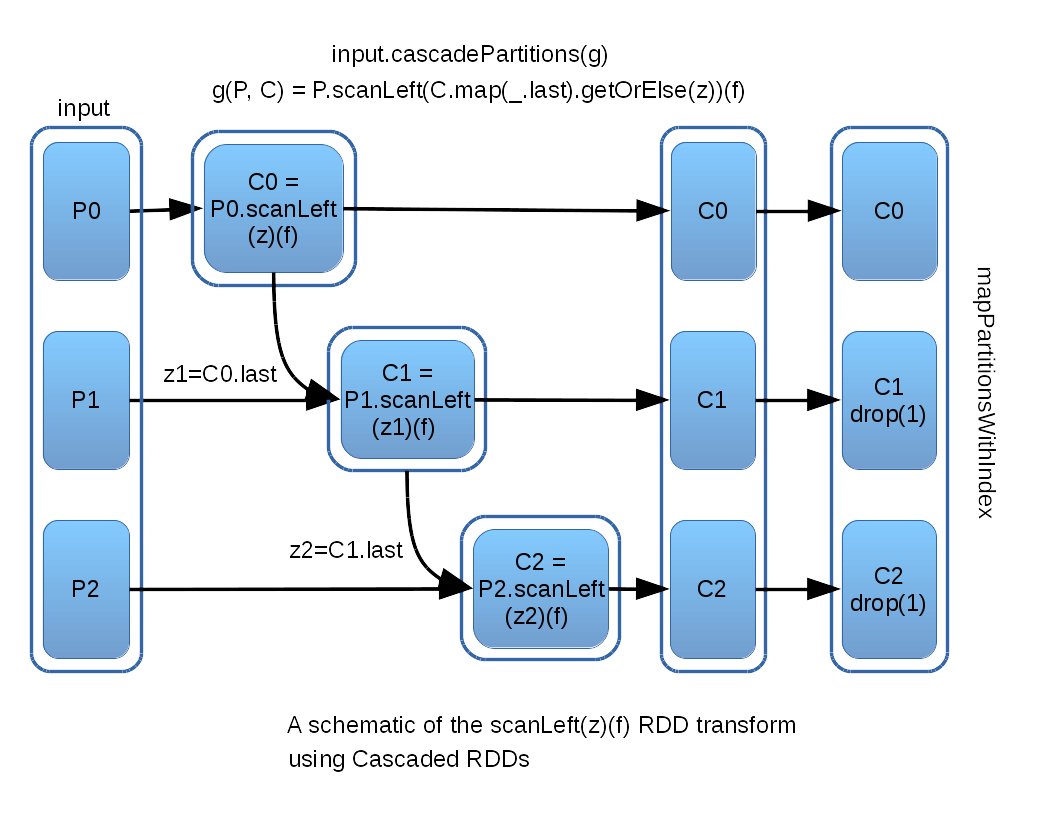In Scala, sequence (and iterator) data types support the scanLeft method for computing a sequential prefix scan on sequence elements:

// Use scanLeft to compute the cumulative sum of some integers
scala> List(1, 2, 3).scanLeft(0)(_ + _)
res0: List[Int] = List(0, 1, 3, 6)


Spark RDDs are logically a sequence of row objects, and so scanLeft is in principle well defined on RDDs. In this post I will describe how to cleanly implement a scanLeft RDD transform by applying an RDD variation called Cascade RDDs.

A Cascade RDD is an RDD having one partition which is a function of an input RDD partition and an optional predecessor Cascade RDD partition. You can see that this definition is somewhat recursive, where the basis case is a Cascade RDD having no precedessor. The following diagram illustrates both cases of Cascade RDD:As implied by the above diagram, a series of Cascade RDDs falling out of an input RDD will have as many Cascade RDDs as there are input partitions. This situation begs for an abstraction to re-assemble the cascade back into a single output RDD, and so the method cascadePartitions is defined, as illustrated:The cascadePartitions method takes a function argument f, with the signature:

f(input: Iterator[T], cascade: Option[Iterator[U]]): Iterator[U]


in a manner somewhat analogous to mapPartitions. The function f must address the fact that cascade is optional and will be None in case where there is no predecessor Cascade RDD. The interested reader can examine the details of how the CascadeRDD class and its companion method cascadePartitions are implemented here.

With Cascade RDDs it is now straightforward to define a scanLeft transform for RDDs. We wish to run scanLeft on each input partition, with the condition that we want to start where the previous input partition left off. The Scala scanLeft function makes this easy, as the starting point is its first parameter (z): scanLeft(z)(f). The following figure illustrates what this looks like:As the above schematic demonstrates, almost all the work is accomplished with a single call to cascadePartitions, using a thin wrapper around f which determines where to start the next invocation of Scala scanLeft – either the input parameter z, or the last output element of the previous cascade. One final transform must be applied to remove the initial element that Scala scanLeft inserts into its output, excepting the first output partition, where it is kept to be consistent with the scanLeft definition.

All computation is accomplished in the standard RDD formalism, and so scanLeft is a proper lazy RDD transform.

The actual implementation is as compact as the above description implies, and you can see the code here.